Next: Logic of Knowledge Up: DISCUSSION OF LITERATURE Previous: DISCUSSION OF LITERATURE

## Modal Logic

It is difficult to give a concise definition of modal logic. It was originally invented by Lewis (1918) in an attempt to avoid the `paradoxes' of implication (a false proposition implies any proposition). The idea was to distinguish two sorts of truth: necessary truth and mere contingent truth. A contingently true proposition is one which, though true, could be false. This is formalized by introducing the modal operator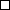(read `necessarily') which forms propositions from propositions. Then p's being a necessary truth is expressed by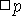's being true. More recently, modal logic has become a much-used tool for analyzing the logic of such various propositional operators as belief, knowledge and tense.

There are very many possible axiomatizations of the logic ofnone of which seem more intuitively plausible than many others. A full account of the main classical systems is given by Feys (1965), who also includes an excellent bibliography. We shall give here an axiomatization of a fairly simple modal logic, the system M of Feys - von Wright. One adds to any full axiomatization of propositional calculus the following: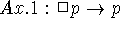.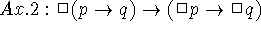.

Rule 1: from p and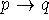, infer q.

Rule 2: from p, infer.

(This axiomatization is due to Gödel.) There is also a dual modal operator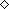, defined as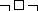. Its intuitive meaning is `possibly':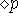is true when p is at least possible, although p may be in fact false (or true). The reader will be able to see the intuitive correspondence between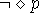--p is impossible, and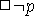--that is, p is necessarily false.

M is a fairly weak modal logic. One can strengthen it by adding axioms, for example, adding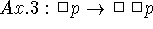yields the system called S4; adding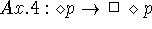yields S5; and other additions are possible. However, one can also weaken all the systems in various ways, for instance by changing Ax. 1 to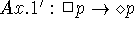. One easily sees that Ax. 1 implies Ax. 1', but the converse is not true. The systems obtained in this way are known as the deontic versions of the systems. These modifications will be useful later when we come to consider tense-logics as modal logics.

One should note that the truth or falsity ofis not decided by p's being true. Thusis not a truth-functional operator (unlike the usual logical connectives, for instance) and so there is no direct way of using truth-tables to analyze propositions containing modal operators. In fact the decision problem for modal propositional calculi has been quite nontrivial. It is just this property which makes modal calculi so useful, as belief, tense, etc., when interpreted as propositional operators, are all nontruthfunctional.

The proliferation of modal propositional calculi, with no clear means of comparison, we shall call the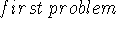of modal logic. Other difficulties arise when we consider modal predicate calculi, that is, when we attempt to introduce quantifiers. This was first done by Barcan-Marcus (1946).

Unfortunately, all the early attempts at modal predicate calculi had unintuitive theorems (see for instance Kripke 1963a), and, moreover, all of them met with difficulties connected with the failure of Leibniz' law of identity, which we shall try to outline. Leibniz' law is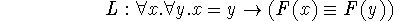where F is any open sentence. Now this law fails in modal contexts. For instance, consider this instance of L: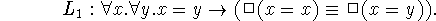By rule 2 of M (which is present in almost all modal logics), since x=x is a theorem, so is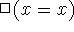. Thus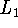yields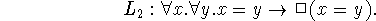But, the argument goes, this is counterintuitive. For instance the morning star is in fact the same individual as the evening star (the planet Venus). However, they are not necessarily equal: one can easily imagine that they might be distinct. This famous example is known as the `morning star paradox'.

This and related difficulties compel one to abandon Leibniz' law in modal predicate calculi, or else to modify the laws of quantification (so that it is impossible to obtain the undesirable instances of universal sentences such as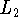). This solves the purely formal problem, but leads to severe difficulties in interpreting these calculi, as Quine has urged in several papers (cf. Quine 1964).

The difficulty is this. A sentence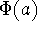is usually thought of as ascribing some property to a certain individual a. Now consider the morning star; clearly, the morning star is necessarily equal to the morning star. However, the evening star is not necessarily equal to the morning star. Thus, this one individual--the planet Venus--both has and does not have the property of being necessarily equal to the morning star. Even if we abandon proper names the difficulty does not disappear: for how are we to interpret a statement like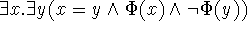?

Barcan-Marcus has urged an unconventional reading of the quantifiers to avoid this problem. The discussion between her and Quine in Barcan-Marcus (1963) is very illuminating. However, this raises some difficulties--see Belnap and Dunn (1968)--and the recent semantic theory of modal logic provides a more satisfactory method of interpreting modal sentences.

This theory was developed by several authors (Hintikka 1963, 1967a; Kanger 1957; Kripke 1963a, 1963b, 1965), but chiefly by Kripke. We shall try to give an outline of this theory, but if the reader finds it inadequate he should consult Kripke (1963a).

The idea is that modal calculi describe several possible worlds at once, instead of just one. Statements are not assigned a single truth-value, but rather a spectum of truth-values, one in each possible world. Now, a statement is necessary when it is true in all possible worlds--more or less. Actually, in order to get different modal logics (and even then not all of them) one has to be a bit more subtle, and have a binary relation on the set of possible worlds--the alternativeness relation. Then a statement is necessary in a world when it is true in all alternatives to that world. Now it turns out that many common axioms of modal propositional logics correspond directly to conditions of alternativeness. Thus for instance in the system M above, Ax. 1 corresponds to the reflexiveness of the alternativeness relation;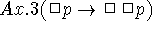corresponds to its transitivity. If we make the alternativeness relation into an equivalence relation, then this is just like not having one at all; and it corresponds to the axiom: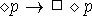.

This semantic theory already provides an answer to the first problem of modal logic: a rational method is available for classifying the multitude of propositional modal logics. More importantly, it also provides an intelligible interpretation for modal predicate calculi. One has to imagine each possible world as having a set of individuals and an assignment of individuals to names of the language. Then each statement takes on its truth value in a world s according to the particular set of individuals and assignment associated with s. Thus, a possible world is an interpretation of the calculus, in the usual sense.

Now, the failure of Leibniz' law is no longer puzzling, for in one world the morning star--for instance--may be equal to (the same individual as) the evening star, but in another the two may be distinct.

There are still difficulties, both formal--the quantification rules have to be modified to avoid unintuitive theorems (see Kripke, 1963a, for the details)--and interpretative: it is not obvious what it means to have the same individual existing in different worlds.

It is possible to gain the expressive power of modal logic without using modal operators by constructing an ordinary truth-functional logic which describes the multiple-world semantics of modal logic directly. To do this we give every predicate an extra argument (the world-variable; or in our terminology the situation-variable) and instead of writing `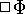', we write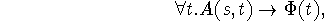where A is the alternativeness relation between situations. Of course we must provide appropriate axioms for A.

The resulting theory will be expressed in the notation of the situation calculus; the proposition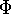has become a propositional fluent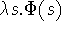, and the `possible worlds' of the modal semantics are precisely the situations. Notice, however, that the theory we get is weaker than what would have been obtained by adding modal operators directly to the situation calculus, for we can give no translation of assertions such as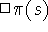, where s is a situation, which this enriched situation calculus would contain.

It is possible, in this way, to reconstruct within the situation calculus subtheories corresponding to the tense-logics of Prior and to the knowledge logics of Hintikka, as we shall explain below. However, there is a qualification here: so far we have only explained how to translate the propositional modal logics into the situation calculus. In order to translate quantified modal logic, with its difficulties of referential opacity, we must complicate the situation calculus to a degree which makes it rather clumsy. There is a special predicate on individuals and situations--exists(i,s)--which is regarded as true when i names an individual existing in the situation s. This is necessary because situations may contain different individuals. Then quantified assertions of the modal logic are translated according to the following scheme: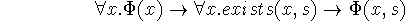where s is the introduced situation variable. We shall not go into the details of this extra translation in the examples below, but shall be content to define the translations of the propositional tense and knowledge logics into the situation calculus.Next: Logic of Knowledge Up: DISCUSSION OF LITERATURE Previous: DISCUSSION OF LITERATURE

John McCarthy
Mon Apr 29 19:20:41 PDT 1996